×#### Thank you for registering.

One of our academic counsellors will contact you within 1 working day.

Click to Chat

1800-1023-196

+91-120-4616500

CART 0

• 0

MY CART (5)

Use Coupon: CART20 and get 20% off on all online Study Material

ITEM
DETAILS
MRP
DISCOUNT
FINAL PRICE
Total Price: Rs.

There are no items in this cart.
Continue Shopping• Complete JEE Main/Advanced Course and Test Series
• OFFERED PRICE: Rs. 15,900
• View Details

```Chapter 3: Squares and Square Roots Exercise – 3.3

Question: 1

Find the squares of the following numbers using column method. Verify the result finding the square using the usual multiplication.

(i) 25

(ii) 37

(iii) 54

(iv) 71

(v) 96

Solution:

Here a = 2, b = 5

Step: 1 Make 3 columns and write the values of a2, 2 x a x b, and b2 in these columns.

Sol.

(i) 25

Here a = 2, b = 5

Step: 1 Make 3 columns and write the values of a2, 2 x a x b, and b2 in these columns.

Column I
Column II
Column III

a2
2 x a x b
b2

4
20
25

Step: 2 Underline the unit digit of b2 (in Column III) and add its tens digit, if any, with 2 x a x b (in column II)

Column I
Column II
Column III

a2
2 x a x b
b2

4
20 + 2
25

22

Step: 3 Underline the unit digit in Column II and add the number formed by the tens and other digits if any, with a2 in Column I.

Column I
Column II
Column III

a2
2 x  a x b
b

4 + 2
20 + 2
25

6
22

Step 4: Underline the number in Column I.

Column I
Column II
Column III

a2
2 x a x b
b2

4 + 2
20 + 2
25

6
22

Step: 5 write the underlined digits at the bottom of each column to obtain the square of the given number.

In this case, we have:

252 = 625

Using Multiplication:

25 x 25 = 625

This matches with the result obtained by the column method:

(ii) 37

Here, a = 3, b = 7

Step: 1 Make 3 columns and write the values of a2, 2 x a x b, and b2 in these columns.

Column I
Column II
Column III

a2
2 x a x b
b2

9
42
49

Step: 2 Underline the unit digit of b2 (in Column III) and add its tens digit, if any, with 2 x a x b (in Column II)

Column I
Column II
Column III

A2
2 x a x b
B2

9 + 4
42 + 4
49

13
46

Step: 3 Underline the unit digit in Column II and add the number formed by tens and others digits if any, with a2 in Column I.

Column I
Column II
Column III

A2
2 x a x b
B2

9 + 4
42 + 4
49

13
46

Step 4:  Write the underlined digits at the bottom of each column to obtain the square of the given number.

In this case, we have:

372 = 1369

Using multiplication:

37 x 37 = 1369

This matches with the result obtained using the column method.

(iii) 54

Here, a = 5, b = 4

Step 1: make 3 columns and write the values of a2, 2 x a x b and b2 in these columns.

Column I
Column II
Column III

a2
2 x a x b
b2

25
40

Step: 2 Underline the unit digit of b2 (in Column III) and add its tens digit, if any, with 2x a x b (in Column II)

Column I
Column II
Column III

a2
2 x a x b
b2

25
40 +1
16

41

Step: 3 Underline the digit in Column II and add the number formed by the tens and other digits if any, with a2 in Column I.

Column I
Column II
Column III

a2
2 x a x b
b2

25 + 4
40 + 1
16

29
41

Step: 4 underline the number in Column I.

Column I
Column II
Column III

a2
2 x a x b
b2

25 + 4
40 + 1
16

29
41

Step: 5 write the underlined digits at the bottom of each column to obtain the square of the given number.

In this case, we have:

542 = 2916

Using multiplication:

54 x 54 = 2916

This matches with the result obtained using the column method.

(iv) 71

Here, a = 7, b = 1

Step: 1 Make 3 columns and write the values of a2, 2 x a x b and b2 in these columns.

Column I
Column II
Column III

a2
2 x a x b
b2

49
14
1

Step: 2 Underline the unit digit of b2 (in column III) and add its ten digit, if any with 2 x a x b (in column II)

Column I
Column II
Column III

a2
2 x a x b
b2

49
14 + 0
1

14

Step: 3 Underline the unit digit in Column II and add the number formed by the tens and other digits, if any, with a2 in column I.

Column I
Column II
Column III

a2
2 x a x b
b2

49 + 1
14 + 0
1

50
14

Step: 4 underline the number in column I.

Column I
Column II
Column III

a2
2 x a x b
b2

49 + 1
14 + 0
1

50
14

Step: 5 write the underlined digits at the bottom of each column to obtain the square of the given number:

In this case, we have:

712 = 5041

Using multiplication:

71 x 71 = 5041

This matches with the result obtained using the column method.

(v) 96

Here, a = 9, b = 6

Step: 1 Make 3 columns and write the values of a2, 2 x a x b and b2 in these columns.

Column I
Column II
Column III

a2
2 x a x b
b2

81
108
36

Step: 2 Underline the unit digit of b2 (in column III) and add its tens digit, if any with 2 x a x b (in column II)

Column I
Column II
Column III

a2
2 x a x b
b2

81
108 + 3
36

111

Step: 3 Underline the unit digit in Column II and add the number formed by the tens and other digits if any, with a2 in column I.

Column I
Column II
Column III

a2
2 x a x b
b2

81 + 11
108 + 3
36

92
111

Step: 4 underline the number in Column I

Column I
Column II
Column III

a2
2 x a x b
b2

81 + 11
108 + 3
36

92
111

Step: 5 write the underlined digits at the bottom of each column to obtain the square of the given number.

In this case, we have:

962 = 9216

Using multiplication:

96 x 96 = 2916

This matches with the result obtained using the column method.

Question: 2

Find the squares of the following numbers using diagonal method:

(i) 98

(ii) 273

(iii) 348

(iv) 295

(v) 171

Solution:

(i) 98

∴ 982 = 9604(ii) 273

∴ 2732 = 74529(iii) 348

∴ 3482 = 121104(iv) 295

∴ 2952 = 87025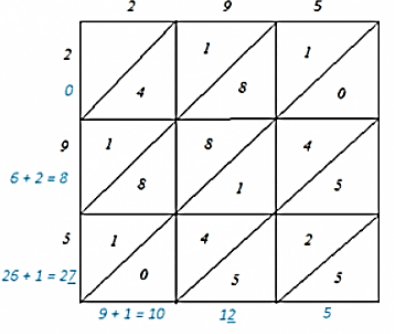(v) 171

∴ 1712 = 29241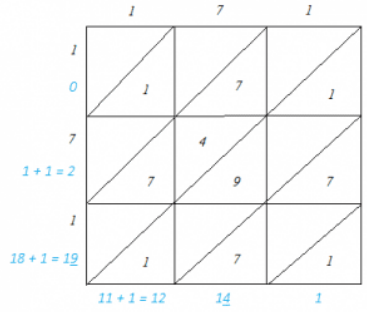Question: 3

Find the squares of the following numbers:

(i) 127

(ii) 503

(iii) 451

(iv) 862

(v) 265

Solution:

We will use visual method as it is the efficient method to solve this problem.

(i) We have:

127 = 120 + 7

Hence, let us draw a square having side 127 units. Let us split it into 120 units and 7 units.Hence, the square of 127 is 16129.

(ii) We have:

503 = 500 + 3

Hence, let us draw a square having side 503 units. Let us split it into 500 units and 3 units.Hence, the square of 503 is 253009.

(iii) We have:

451 = 450 + 1

Hence, let us draw a square of having side 451 units. Let us split it into 450 units and 1 units.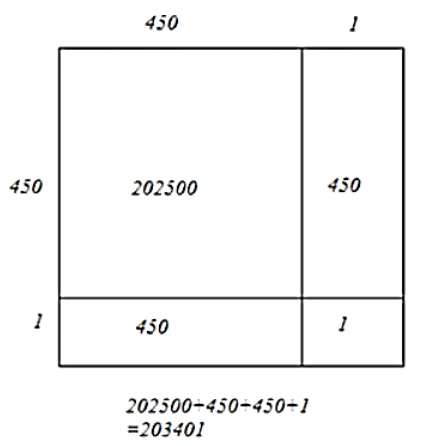Hence, the square of 451 is 203401.

(iv) We have:

862 = 860 + 2

Hence, let us draw a square having side 862 units. Let us split it into 860 units and 2 units.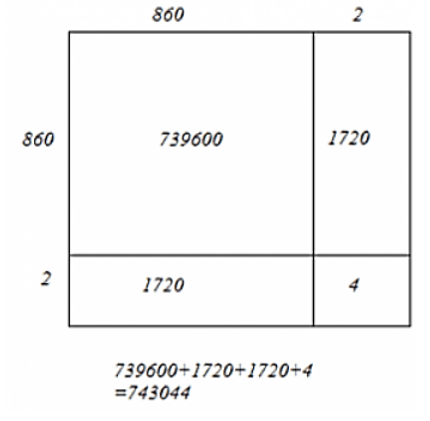Hence, the square of 862 is 743044.

(v) We have:

265 = 260 +5

Hence, let us draw a square having 265 units. Let us split it into 260 units and 5 units.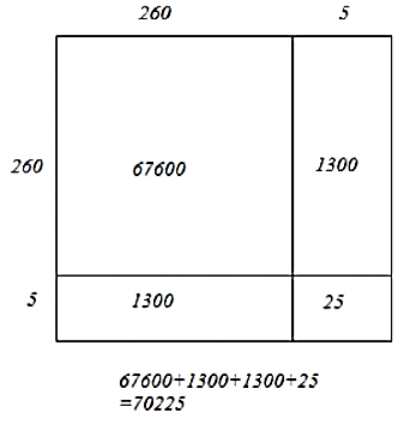Hence, the square of 265 units is 70225.

Question: 4

Find the squares of the following numbers:

(i)  425

(ii) 575

(iii) 405

(iv) 205

(v) 95

(vi) 745

(vii) 512

(viii)  995

Notice that all numbers except the one in question (vii) has 5 as their respective unit digits. We know that the square of a number with the form n5 is a number ending with 25 and has the number n(n + 1) before 25.

Solution:

Sol.

(i)  425

Here, n = 42

∴ n(n + 1) = (42)(43) = 1806

∴ 4252 = 180625

(ii) 575

Here, n = 57

∴n(n + 1) = (57)(58) = 3306

∴ 5752 = 330625

(iii) 405

Here n = 40

∴n(n + 1) = (40)(41) = 1640

∴ 4052 = 164025

(iv) 205

Here n = 20

∴n(n + 1) = (20)(21) = 420

∴ 2052 = 42025

(v) 95

Here n = 9

∴ n(n + 1) = (9)(10) = 90

∴ 952 = 9025

(vi) 745

Here n = 74

∴ n(n + 1) = (74)(75) = 5550

∴ 7452 = 555025

(vii) 512

We know: The square of a three-digit number of the form 5ab = (250 + ab) 1000 + (ab)2

∴ 5122 = (250+12)1000 + (12)2 = 262000 + 144 = 262144

(viii)  995

Here, n = 99

∴ n(n + 1) = (99)(100) = 9900

∴ 9952 = 990025

Question: 5

Find the squares of the following numbers using the identity (a +b)2 = a2 + 2ab + b2:

(i) 405

(ii) 510

(iii) 1001

(iv) 209

(v) 605

Solution:

(i) 405

On decomposing:

405 = 400 + 5

Here, a = 400x and b = 5

Using the identity (a + b)2 = a2 + 2ab + b2:

4052 = (400 + 5)2= 4002 + 2(400)(5) + 52 = 160000 + 4000 + 25 = 164025

(ii) 510

On decomposing:

510 = 500 + 10 Here, a = 500 and b = 10

Using the identity (a + b)2 = a2 + 2ab + b2:

5102 = (500 + 10)2 = 5002 + 2(500)(10) + 102 = 250000 + 10000 + 100 = 260100

(iii) 1001

On decomposing:

1001 = 1000 + 1

Here, a = 1000 and b = 1

Using the identity (a + b)2 = a2 + 2ab + b2:

10012 = (1000 +1)2 = 10002 + 2(1000) (1) + 12 = 1000000 + 2000 + 1 = 1002001

(iv) 209

On decomposing:

209 = 200 + 9

Here, a = 200 and b = 9

Using the identity (a + b) 2 = a2+ 2ab + b2:

2092 = (200 + 9)2 = 2002 + 2(200)(9) + 92 = 40000 + 3600 + 81 = 43681

(v) 605

On decomposing:

605 = 600 + 5

Here, a = 600 and b = 5

Using the identity (a + b)2 = a2 + 2ab + b2:

6052 = (600 + 5)2 = 6002 + 2(600)(5) + 52 = 360000 + 6000 + 25 = 366025

Question: 6

Find the squares of the following numbers using the identity (a – b)2 = a2  – 2ab + b2:

(i) 395

(ii) 995

(iii) 495

(iv) 498

(v) 99

(vi) 999

(vii) 599

Solution:

(i) 395

Decomposing: 395 = 400 - 5

Here, a = 400 and b = 5

Using the identity (a — b) 2 = a2 – 2ab + b2:

3952 = (400 - 5)2 = 4002 - 2(400)(5) + 52 = 160000 - 4000 + 25 = 156025

(ii) 995

Decomposing:

995 = 1000 - 5

Here, a =1000 and b = 5

Using the identity (a -b) 2 = a2 - 2ab + b2:

9952 = (1000 - 5)2 = 10002 -2(1000)(5) + 52 = 1000000 -10000 + 25 = 990025

(iii) 495

Decomposing: 495 = 500 - 5 Here, a = 500 and b = 5 Using the identity (a - b) 2 = a2 - 2ab + b2:

4952 = (500 - 5)2 = 5002 - 2(500) (5) + 52 = 250000 - 5000 + 25 = 245025

(iv) 498

Decomposing: 498 = 500 - 2 250000 - 5000 + 25 = 245025

Here, a = 500 and b = 2

Using the identity (a - b)2 = a2 - 2ab + b2:

4982 = (500 -2)2 = 5002 -2(500)(2) + 22 = 250000 - 2000 + 4 = 248004

(v) 99

Decomposing: 99 = 100 -1 Here, a = 100 and b = 1 Using the identity (a - b)2 = a2 - 2ab + b2: 992 = (100-1)2 = 1002- 2(100)(1) + 12 = 10000 -200 + 1 = 9801

(vi) 999

Decomposing: 999 = 1000 – 1

Here, a = 1000 and b = 1

Using the identity (a - b)2 = a2 - 2ab + b2:

9992 = (1000 – 1)2 – 10002 – 2(1000) (1) + 12 = 1000000 – 2000 +1 = 998001

(vii) 599

Decomposing: 599 = 600 -1

Here, a = 600 and b = 1

Using the identity (a - b) 2 = a2 -2ab + b2:

5992 = (600 -1)2 = 6002-2(600) (1) + 12 = 360000 -1200 + 1 = 358801

Question: 7

Find the squares of the following numbers by visual method:

(i) 52

(ii) 95

(iii) 505

(iv) 702

(v) 99

Solution:

(i) 52

We have:

52 = 50 + 2

Let us draw a square having side 52 units. Let us split it into 50 units and 2 units.The sum of the areas of these four parts is the square of 52. Thus, the square of 52 is 2704.

(ii) 95

We have:

95 = 90 + 5

Let us draw a square having side 95 units. Let us split it into 90 units and 5 units.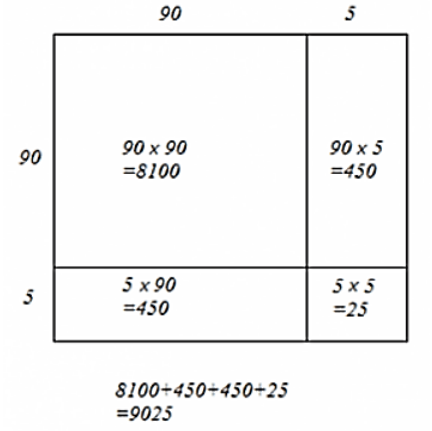The sum of the areas of these four parts is the square of 95. Thus, the square of 95 is 9025.

(iii) 505

We have:

505 = 500 + 5

Let us draw a square having side 505 units. Let us split it into 500 units and 5 units.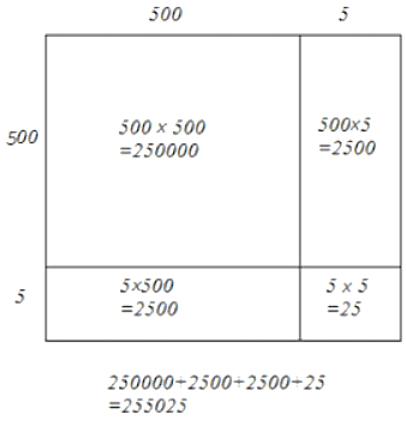The sum of the areas of these four parts is the square of 505. Thus, the square of 505 is 255025.

(iv) 702

We have:

702 = 700 + 2

Let us draw a square of having side 702 units. Let us split it into 700 units and 2 units.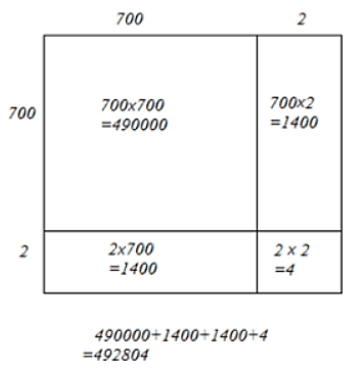The sum of the areas of these four parts is the square of 702. Thus, the square of 702 is 492804.

(v) 99

We have:

99 = 90 + 9

Let us draw a square of having side 99 units. Let us split it into 90 units and 9 units.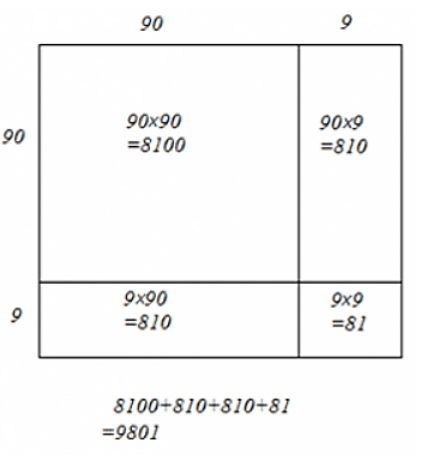The sum of the areas of these four parts is the square of 99. Thus, the square of 99 is 9801.
```### Course Features

• 728 Video Lectures
• Revision Notes
• Previous Year Papers
• Mind Map
• Study Planner
• NCERT Solutions
• Discussion Forum
• Test paper with Video Solution